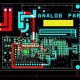# Interview Questions for Rectifier Circuit

In this tutorial we will learn about interview Questions for Rectifier Circuit

Question 1. What is Rectifier?

Answer – It is a device which converts alternating current (or voltage) into unidirectional current (or voltage)

Question 2. What is a DC Power Supply?

Answer – Which device that converts to AC into DC is called DC Power Supply.

Question 3. How many types of rectifier?

Answer – Three of rectifier 1. Half wave rectifier 2. Full wave centre – tapped rectifier

3. Full wave bridge rectifier.

Question 4. What is meaning of PIV of a diode in a rectifier circuit?

Answer – In diode PIV means Peak inverse voltage, it is maximum possible voltage that occurs across a diode when it is reverse biased.

Question 5. What is filter in rectifier circuit?

Answer – A filter in rectifier circuit that converts the pulsating output of the rectifier into steady DC level.

Question 6. Why don’t use series inductor and L section filters cannot be used with half wave rectifier?

Answer – In half wave rectifier series inductor and L section filter cannot be used with half wave rectifier because an operation of series inductor depends upon the current through  and also it needs a minimum current to flow at all times.

Question 7. What is mean by voltage regulation of a DC power supply?

Answer – Its means the change in voltage from no load to the full load condition is known as voltage regulation.

Question 8. What is the use of bleeder resistance in a rectifier circuit using L-C filter?

Answer – In rectifier of circuit where bleeder resistance is placed in parallel with load so as to maintain a certain minimum current through the choke, even the load resistor gets open circuited and improves filtering action.

Question 9. Why use R-C filter only in Light loads?

Answer – R-C filter have poor voltage regulation and it also generate heat through R.

So that it is use only for light loads.

Question 10. What is utilization factor in transformer?

Answer – Utilization factor is defined as the ratio of power delivered to the load and a rating of a secondary supply power transformer.

Question 11. If full wave rectifier of output is 60HZ then ripple is also 60HZ then what is circuit reaction?

Answer – A full wave rectifier with 60HZ input must have the lowest ripple frequency equal to twice the input frequency example – 120HZ, if the ripple frequency is 60HZ.it means some diodes are not working in circuit.

Question 12. Why the capacitor input filter is preferred to choke input filter?

Answer – In the capacitor input filter, the DC output is much larger and ripples are less in comparison to those in choke input filter, so the capacitor input filter.

Question 13.  Are  – Filters  suitable for varying loads?

Answer – Voltage regulation in case of  filter is very poor and, therefore,  filter is not suitable for varying loads.

Question 14.  Are Diodes  operated in the breakdown region in rectifiers?

Answer – In the breakdown region, a diode has a risk of getting damaged or burnt because the magnitude of the current flowing through it increases in an in controlled manner. That’s why diodes are not operated in the breakdown region in rectifiers.

Question 15. What ripple is as referred to in a rectifier circuit.

Answer – The AC components contained in the pulsating output of a rectifier is known as ripple.